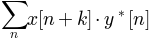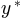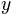/Matplotlib 3.0

# matplotlib.pyplot.xcorr

`matplotlib.pyplot.xcorr(x, y, normed=True, detrend=<function detrend_none>, usevlines=True, maxlags=10, *, data=None, **kwargs)` [source]

Plot the cross correlation between x and y.

The correlation with lag k is defined as, whereis the complex conjugate of.

Parameters: `x : sequence of scalars of length n` `y : sequence of scalars of length n` `detrend : callable, optional, default: mlab.detrend_none` x is detrended by the detrend callable. Default is no normalization. `normed : bool, optional, default: True` If `True`, input vectors are normalised to unit length. `usevlines : bool, optional, default: True` If `True`, `Axes.vlines` is used to plot the vertical lines from the origin to the acorr. Otherwise, `Axes.plot` is used. `maxlags : int, optional` Number of lags to show. If None, will return all `2 * len(x) - 1` lags. Default is 10. `lags : array (length 2*maxlags+1)` lag vector. `c : array (length 2*maxlags+1)` auto correlation vector. `line : LineCollection or Line2D` `Artist` added to the axes of the correlation `LineCollection` if usevlines is True `Line2D` if usevlines is False `b : Line2D or None` Horizontal line at 0 if usevlines is True None usevlines is False `linestyle : Line2D property, optional` Only used if usevlines is `False`. `marker : string, optional` Default is 'o'.

#### Notes

The cross correlation is performed with `numpy.correlate()` with `mode = 2`.

Note

In addition to the above described arguments, this function can take a data keyword argument. If such a data argument is given, the following arguments are replaced by data[<arg>]:

• All arguments with the following names: 'x', 'y'.

Objects passed as data must support item access (`data[<arg>]`) and membership test (`<arg> in data`).